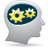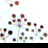# How to capture the Console in debugging a JTAG configuration issue

cancel
Showing results for
Did you mean:
SOLVED

## How to capture the Console in debugging a JTAG configuration issue

600 ViewsContributor III

I'm trying to debug an issue where "CCSProtocolPlugin : Failed to correctly configure the JTAG chain" even though i've run the CCS IDcode.tcl and ir commands in the server and the chain looks fine. My JTAG chain text file matches the CW JTAG file format, i've even used the processor expert to output the file i need and it still doesn't work. I've enabled logging so i can catch where it fails in the console output but the console deletes everything automatically when it fails. I changed the settings so the console would output to a file but the file is always blank. How can i capture the log? I've tried selecting all and copying as fast as i can and what i get is pasted below. I'm trying to connect to a T2080 with the CodeWarrior TAP over USB.

I've done this multiple times, it appears to be normal that the connection is refused one or two times at first before it continues. It writes all of the RCW registers and appears to fail when resetting.....or at least that's what i could capture.

ccs_open

port = 41475

timeout = 15

serverh = 0

ccs_open; ccs_error = 10

Error message: Connection refused

ccs_open

port = 41475

timeout = 15

serverh = 0

ccs_open; ccs_error = 10

Error message: Connection refused

ccs_open

port = 41475

timeout = 15

serverh = 0

ccs_open; ccs_error = 0

ccs_get_connection_count

serverh = 0

count = 1

ccs_get_connection_count; ccs_error = 0

ccs_available_connections

serverh = 0

count = 0

ccs_available_connections; ccs_error = 0

ccs_available_connections

serverh = 0

count = 0

ccs_available_connections; ccs_error = 0

ccs_config_cc

serverh = 0

config_string = cwtap:0

ccs_config_cc; ccs_error = 0

ccs_available_connections

serverh = 0

count = 1

ccs_available_connections; ccs_error = 0

ccs_cc_version

serverh = 0

cc = 0

version.major = 0

version.minor = 0

ccs_cc_version; ccs_error = 0

ccs_set_timeout

serverh = 0

timeout = 15

ccs_set_timeout; ccs_error = 0

ccs_available_connections

serverh = 0

count = 1

ccs_available_connections; ccs_error = 0

ccs_config_server

serverh = 0

cc = 0

server_config = 0

value = 10230

ccs_config_server; ccs_error = 0

ccs_get_config_chain

serverh = 0

device_list: (size = 0)

ccs_get_config_chain; ccs_error = 0

ccs_config_chain

serverh = 0

cc = 0

device_list: (size = 1)

device:: core_type=T2080(242)

ccs_config_chain; ccs_error = 0

ccs_config_template

coreh = [serverh:0;cc_index:0;chain_pos:0]

config_reg = 0

config_data = 500

ccs_config_template; ccs_error = 0

ccs_config_template

coreh = [serverh:0;cc_index:0;chain_pos:0]

config_reg = 2

config_data = 1

ccs_config_template; ccs_error = 0

ccs_write_register

coreh = [serverh:0;cc_index:0;chain_pos:0]

index = 210001

count = 1

size = 4

value: (size = 4)

0C00100F

ccs_write_register; ccs_error = 0; duration=0 ms

ccs_write_register

coreh = [serverh:0;cc_index:0;chain_pos:0]

index = 210002

count = 1

size = 4

value: (size = 4)

05000000

ccs_write_register; ccs_error = 0; duration=0 ms

ccs_write_register

coreh = [serverh:0;cc_index:0;chain_pos:0]

index = 210003

count = 1

size = 4

value: (size = 4)

00000000

ccs_write_register; ccs_error = 0; duration=16 ms

ccs_write_register

coreh = [serverh:0;cc_index:0;chain_pos:0]

index = 210004

count = 1

size = 4

value: (size = 4)

00000000

ccs_write_register; ccs_error = 0; duration=0 ms

ccs_write_register

coreh = [serverh:0;cc_index:0;chain_pos:0]

index = 210005

count = 1

size = 4

value: (size = 4)

6C2D0002

ccs_write_register; ccs_error = 0; duration=0 ms

ccs_write_register

coreh = [serverh:0;cc_index:0;chain_pos:0]

index = 210006

count = 1

size = 4

value: (size = 4)

F0006A00

ccs_write_register; ccs_error = 0; duration=0 ms

ccs_write_register

coreh = [serverh:0;cc_index:0;chain_pos:0]

index = 210007

count = 1

size = 4

value: (size = 4)

EC026000

ccs_write_register; ccs_error = 0; duration=0 ms

ccs_write_register

coreh = [serverh:0;cc_index:0;chain_pos:0]

index = 210008

count = 1

size = 4

value: (size = 4)

C1000000

ccs_write_register; ccs_error = 0; duration=0 ms

ccs_write_register

coreh = [serverh:0;cc_index:0;chain_pos:0]

index = 210009

count = 1

size = 4

value: (size = 4)

02180000

ccs_write_register; ccs_error = 0; duration=0 ms

ccs_write_register

coreh = [serverh:0;cc_index:0;chain_pos:0]

index = 210010

count = 1

size = 4

value: (size = 4)

00000001

ccs_write_register; ccs_error = 0; duration=0 ms

ccs_write_register

coreh = [serverh:0;cc_index:0;chain_pos:0]

index = 210011

count = 1

size = 4

value: (size = 4)

00000000

ccs_write_register; ccs_error = 0; duration=15 ms

ccs_write_register

coreh = [serverh:0;cc_index:0;chain_pos:0]

index = 210012

count = 1

size = 4

value: (size = 4)

000197FC

ccs_write_register; ccs_error = 0; duration=2 ms

ccs_write_register

coreh = [serverh:0;cc_index:0;chain_pos:0]

index = 210013

count = 1

size = 4

value: (size = 4)

00000100

ccs_write_register; ccs_error = 0; duration=2 ms

ccs_write_register

coreh = [serverh:0;cc_index:0;chain_pos:0]

index = 210014

count = 1

size = 4

value: (size = 4)

5280000D

ccs_write_register; ccs_error = 0; duration=2 ms

ccs_write_register

coreh = [serverh:0;cc_index:0;chain_pos:0]

index = 210015

count = 1

size = 4

value: (size = 4)

00000000

ccs_write_register; ccs_error = 0; duration=2 ms

ccs_write_register

coreh = [serverh:0;cc_index:0;chain_pos:0]

index = 210016

count = 1

size = 4

value: (size = 4)

00000004

ccs_write_register; ccs_error = 0; duration=2 ms

ccs_reset_to_debug

serverh = 0

cc = 0

Labels (1)
• ### General

1 Solution
261 ViewsContributor III

I'm new to this environment so i didn't realize that their are multiple console screens and you have to switch back to your logging console to see it.

2 Replies
262 ViewsContributor III

I'm new to this environment so i didn't realize that their are multiple console screens and you have to switch back to your logging console to see it.

261 ViewsNXP Employee

Hi,

I think when you remove the termination, the log will show again on the console. No?

Regards

Lunmin